# The SSM Procedure

#### Multivariate Random Walk Trend

The STATE statement option TYPE=RW specifies a dim-dimensional random walk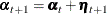where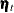is a sequence of zero mean, independent, Gaussian vectors with covariance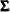. The specification of the associated system matrices is trivial: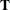is a dim-dimensional identity matrix,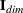,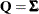, and the initial condition is fully diffuse (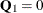and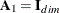).

The multivariate random walk is a useful trend model for multivariate time series data. The trend term for the ith response variable is defined by a component that simply picks the ith (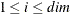) element of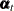. For example, the component rw_i defined as follows can be used as a trend term in the MODEL statement of the ith response variable:

     state randomWalk(3) type=rw ...;
component rw_2 = randomWalk;